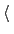Next: Adaptive Linear Bias/Experiment Directed Up: Biasing and analysis methods Previous: Harmonic restraints   Contents   Index

## Linear restraints

The linear restraint biasing method is used to minimally bias a simulation. There is generally a unique strength of bias for each CV center, which means you must know the bias force constant specifically for the center of the CV. This force constant may be found by using experiment directed simulation described in section 13.5.6. Please cite Pitera and Chodera when using .

• name: see definition of name (biasing and analysis methods)
• colvars: see definition of colvars (biasing and analysis methods)

• forceConstantScaled force constant (kcal/mol)Context: linear
Acceptable values: positive decimal
Default value: 1.0
Description: This defines a scaled force constant for the linear bias. To ensure consistency for multidimensional restraints, it is divided internally by the specific width for each colvar involved (which is 1 by default), so that all colvars are effectively dimensionless and of commensurate size.

• centersInitial linear restraint centersContext: linear
Acceptable values: space-separated list of colvar values
Description: The centers (equilibrium values) of the restraint are entered here. The number of values must be the number of requested colvars. Each value is a decimal number if the corresponding colvar returns a scalar, a (x, y, z)'' triplet if it returns a unit vector or a vector, and a q0, q1, q2, q3)'' quadruplet if it returns a rotational quaternion. If a colvar has periodicities or symmetries, its closest image to the restraint center is considered when calculating the linear potential.Next: Adaptive Linear Bias/Experiment Directed Up: Biasing and analysis methods Previous: Harmonic restraints   Contents   Index
vmd@ks.uiuc.edu